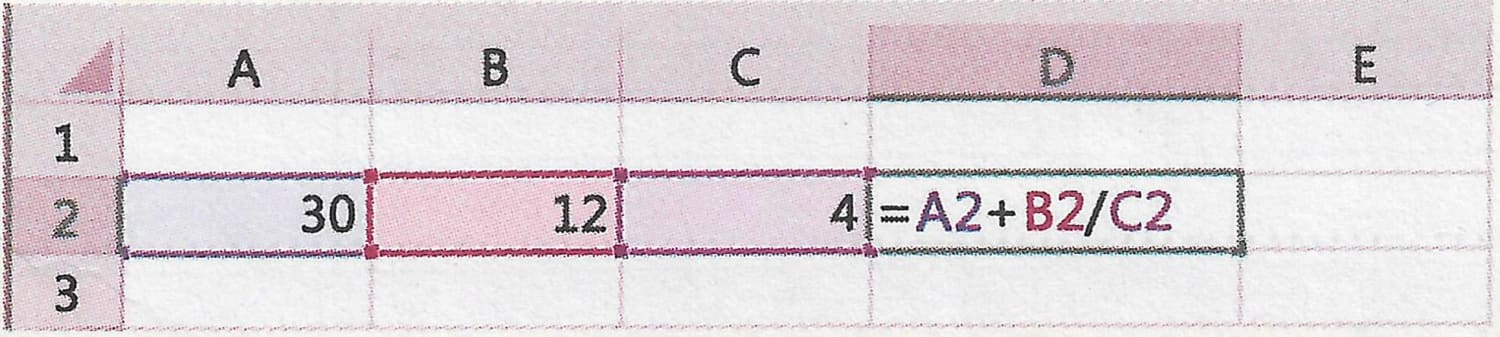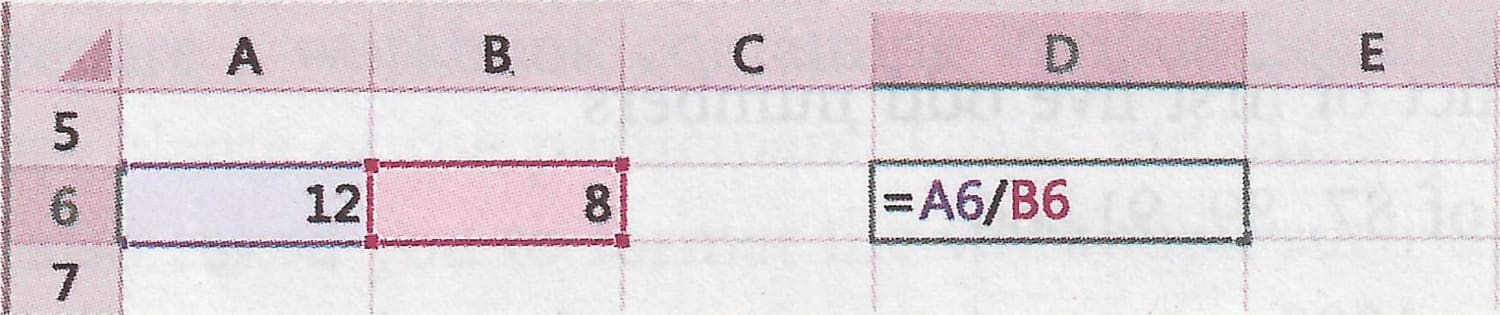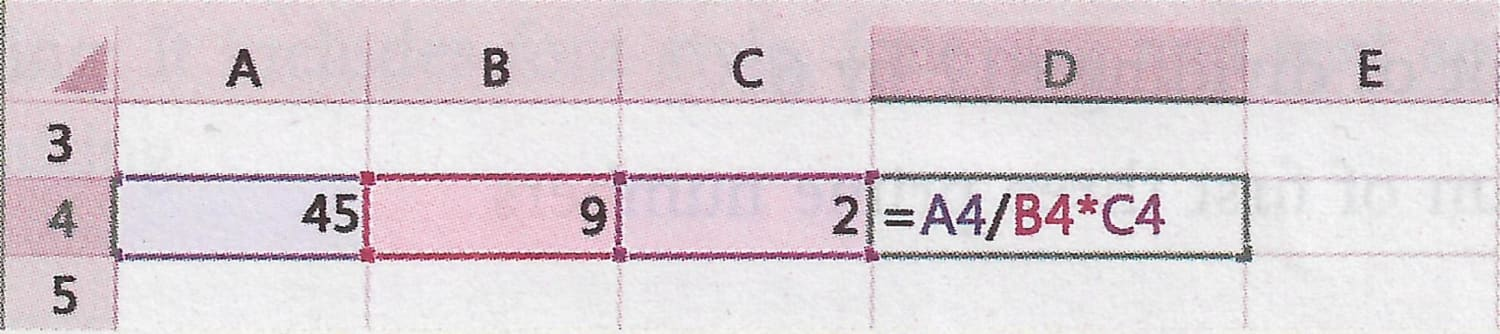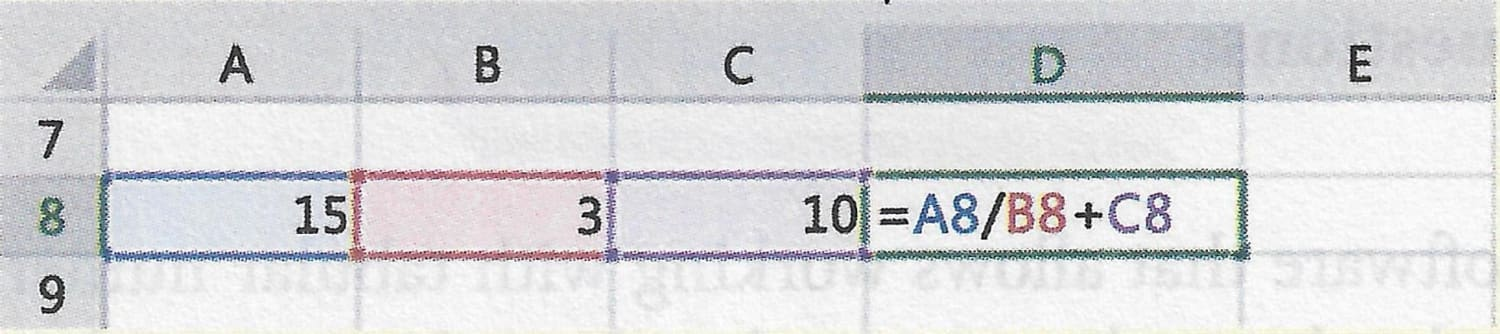## Choose the correct option

#### Question 1

Which of the following is formed due to the intersection of a row and a column?

1. Worksheet
2. Sheet 1
3. Range
4. Cell

Cell

Reason — A cell is the smallest unit of a worksheet, which is formed at the intersection of a row and a column.

#### Question 2

Which is the default worksheet in a workbook?

1. Sheet 1
2. Sheet 3
3. Sheet 1 to Sheet 3
4. Active worksheet

Sheet 1

Reason — Whenever a new workbook is opened its first sheet is named Sheet 1, hence, Sheet 1 is the default worksheet in a workbook.

#### Question 3

Which is the next column followed by column Z?

1. A
2. AA
3. AB
4. ZA

AA

Reason — After A to Z, the column naming starts as AA, AB, AC and so on.

#### Question 4

In which cell, the value entered from the keyboard will appear in a worksheet?

1. In all the columns of selected row
2. In all the rows of the selected column
3. In the active cell
4. In corner cell

In the active cell

Reason — The value entered from the keyboard will appear in the active cell of the worksheet.

#### Question 5

Which of the following options is used to reverse the last action performed by the user in a worksheet?

1. ctrl+A
2. ctrl+X
3. ctrl+Y
4. ctrl+Z

ctrl+Z

Reason — ctrl+Z is the shortcut key to undo the last action performed by the user in a worksheet.

## Fill in the blanks

#### Question 1

The extension of a workbook in MS Excel is .xlsx by default.

#### Question 2

A file, which contains a number of worksheets is called workbook.

#### Question 3

The smallest unit of a worksheet is called cell.

#### Question 4

A cell is referred as column letter followed by a row number.

#### Question 5

When you click on Print option (in MS Excel 2016) it displays the Print Preview of a worksheet.

## State True or False

#### Question 1

You can't close an active worksheet without saving the cell contents.
False

#### Question 2

You may or may not write the extension of a workbook while saving it.
True

#### Question 3

Columns are the horizontal lines in a worksheet.
False

#### Question 4

A cell E10 means column E and 10th row.
True

#### Question 5

Save As option doesn't ask the file name from the user while saving.
False

## Name the following components of spreadsheet

#### Question 1

It stores few shortcut buttons that are frequently used.

#### Question 2

This button displays all the frequently used tasks related to the application.

File button

#### Question 3

It is used to write formulae to perform different operations.

Formula Bar

#### Question 4

It allows inserting more worksheet in a workbook.

New Sheet

#### Question 5

It is used to write formula to perform different mathematical and logical tasks.

Formula Bar

## Case-Study Based Questions

#### Question 1

Formula is an expression consisting of numeric values and operators. You can perform arithmetical operations on a set of values using formulae. It is important to know that all MS Excel formulae must begin with an equal sign (=) followed by an expression. The formulae used to perform different tasks are shown below:The space is provided alongside to write the result of the formulae for each of the above tasks.

(a) 33
Working — A2 = 30, B2 = 12, C2 = 4
Formula = A2 + B2 / C2
= 30 + 12 / 4
= 30 + 3 = 33

(b) 1.5
Working — A6 = 12, B6 = 8
Formula = A6/B6
= 12 / 8 = 1.5

(c) 10
Working — A4 = 45, B4 = 9, C4 = 2
Formula = A4 / B4 * C4
= 45 / 9 * 2
= 5 * 2 = 10

(d) 15
Working — A8 = 15, B8 = 3, C8 = 10
Formula = A8 / B8 + C8
= 15 / 3 + 10
= 5 + 10 = 15

#### Question 1

Spreadsheet is a large sheet which contains data and information. It is a grid of rows and columns and is also called a worksheet. A computer spreadsheet is also known as Electronic spreadsheet. It is used for analyzing and evaluating data represented in tabular form.

#### Question 2

What is the significance of Undo and Redo buttons?

The Undo feature can quickly correct mistakes that we make in a worksheet. When we want to reverse our last action in a worksheet, we click on 'Undo' button.

The Redo button lets us perform the previous task by using 'Undo'. It changes in response to whatever action we just took. The 'Redo' button becomes active whenever we use 'Undo' button.

#### Question 3

Define the following terms

1. Worksheet
2. Workbook
3. Cell
4. Active cell
5. Formula bar

Worksheet — Each page of a workbook is called worksheet. The active worksheet is displayed in the window. The default worksheets are sheet 1, sheet 2, sheet 3.

Workbook — Workbook is a file, which contains a number of worksheets. By default, three worksheets are present in a workbook. You can add or remove worksheets from a workbook as per the requirement.

Cell — A cell is the smallest unit of the worksheet, which is formed at the intersection of a row and a column. Each cell has its own cell address for reference. The address of the cell consists of the column letter followed by the row number.

For example, Cell F4 means column F and row 4.

Active cell — The cell in which cell pointer is located in a worksheet is the active cell. It has a dark boundary around it. It is also known as the cell. We can move cell pointer to make any other cell active either with the help of arrow keys of the keyboard or by using mouse.

Formula bar — It is used to write formulae to perform different mathematical and logical tasks.

#### Question 1

To find the product of first five odd numbers

``````=(1 * 3 * 5 * 7 * 9)
``````

#### Question 2

To find the sum of 87, 89, 91, 66

``````=(87 + 89 + 91 + 66)
``````

#### Question 3

Subtract 999 from 1000

``````=(1000 - 999)
``````

#### Question 4

To show the result of dividing 612 by 6

``````=(612 / 6)
``````

#### Question 5

To display the sum of first three prime numbers

``````=2+3+5
``````

#### Question 1

To find the sum of cell values A2 to A5

``````=A2+A3+A4+A5
``````

#### Question 2

To subtract the cell value D5 from the sum of cell values D3 and D4

``````=D5-(D4+D3)
``````

#### Question 3

To add cell value B5 with the product of cell values B2 to B4

``````=B5+(B2*B3*B4)
``````

#### Question 4

To divide the sum of cell values of E1 and E2 by E4

``````=(E1+E2)/E4
``````

#### Question 1

1. By default, it creates the arrangement of data into columns and rows called cells.
2. The data that is input into the spreadsheet can be either in the form of numbers, strings or formulae.
3. The inbuilt programs allow you to change the appearance of the spreadsheet, including the column width, row height, font color and color of the spreadsheet very easily.
4. You can choose to work with or print a whole spreadsheet or specify a particular area, called a range.
5. MS Excel spreadsheet enhances to analyze large amounts of data with the help of powerful tools.

#### Question 2

Enlist the features of an electronic spreadsheet.

The features of an electronic spreadsheet are as follows:

1. MS Excel is easy to learn and thus, it does not require any specialised training programme.
2. MS Excel basically provides an electronic spreadsheet where all the calculations can be done automatically through built-in programs.
3. Spreadsheets are required mainly for tabulation of data. It minimizes manual work and provides high degree of accuracy in results.
4. It also provides multiple copies of the spreadsheets.
5. To perfrom the basic Mathematical operations such as addition, subtraction, multiplication or division to produce numeric results, we use arithmetic operators like +,-,*,/.
6. It presents the information in the form of charts and graphics.
7. MS Excel is able to sort the data in either ascending or descending order very easily.

#### Question 3

Write down the main steps to perform the following tasks with reference to MS Excel:

(a) To create a new workbook

(b) To save a workbook

(c) To print a workbook

(a) To create a new workbook

To create a new workbook, follow these steps:

Step 1: Click 'File' tab from the menu bar.

Step 2: Select 'New' from the drop-down list.

Step 3: Select 'Blank Workbook' followed by 'Create' that is located on the right side of the window.

The new workbook will be created and opened.

(b) To save a workbook

To save a workbook, follow these steps:

Step 1: Click 'File' button and a sub menu displays on the screen.

Step 2: Click 'Save As' option from the drop down list. Save As dialog box appears on the screen.

Step 3: Choose the location where you want to save your file.

Step 4: Enter the filename in the box provided.

Step 5: Click 'Save' button.

Thus, the workbook will be saved.

(c) To print a workbook

To print a workbook, follow these steps:

Step 1: Select the file and open it in MS Excel.

Step 2: Click 'File' and then 'Print' from the drop-down menu. The right side of the window area shows the print preview and left adjacent part shows the printing parameters. The different parameters are:

1. Printer — Select the printer that is connected to your system.
2. Settings —
a) Select the option 'Print entire workbook'
b) Specify the page orientation i.e., Portrait or Landscape. Default setting is Portrait.
c) Specify the number of copies required.

Step 3: Finally, click 'Print' button.

The workbook will be printed.

#### Question 4

What are the different types of data used in MS Excel? Explain.

Three frequently used data types in MS Excel are:

1. Number — This data represents numeric values including both integers and real numbers. They take part in all arithmetical operations such as addition, subtraction, multiplication, division, etc. By default, the numbers are right aligned.
For example, 1500, -345, 645.67, -76.50, etc.
2. String — The String type data are also known as text data in MS Excel. They contain alphanumeric characters (i.e. uppercase letters/lowercase letters, digits and special symbols). They don't take part in arithmetical operations. By default, the strings are left aligned.
For example, Name, Marks, Total, Product_Name, Pay2021, etc.
3. Formula — It is an expression consisting of numeric values and operators. You can perform all arithmetical operations on a set of values using formulae. All Excel formulae must begin with an equal sign (=) followed by an expression. Some examples are as illustrated below:
1. To find the sum of two values say, 10 and 20
Formula: =10 + 20
2. To add the cell values from A1 to A3
Formula: =A1 + A2 + A3
3. To add the cell value A3 with the product of cell values A1 and A2
Formula: =A1 * A2 + A3

#### Question 5

How will you perform simple addition and multiplication of numbers in a cell of a worksheet? Explain.

Steps to perform simple addition in a cell of a worksheet:

Step 1: Select the cell (say, B2) where you want to perform the addition.

Step 2: Type the required expression, for example =45 + 32

Step 3: Press Enter key. The result is shown in the selected cell. (For our example, B2 will show the result as 77).

Steps to perform multiplication in a cell of a worksheet:

Step 1: Select the cell (say, C2) where you want to perform the multiplication.

Step 2: Type the required expression, for example =12*10*5

Step 3: Press Enter key. The result is shown in the selected cell. (For our example, C2 will show the result as 600).

#### Question 6

Using the concept of formula in MS Excel, write down all the steps:

(a) to calculate the product of values available in the cells A2, B2, C2 and D2

(b) to find the result when sum of cells A4 and A5 are divided by A6

(a) Steps to calculate the product of values available in the cells A2, B2, C2 and D2:

Step 1: Select the cell (say, E2), where you want to get the resulting value.

Step 2: Type equal sign (=) in the cell (cell E2 as per our example) and write the formula =A2*B2*C2*D2. Cells A2 to D2 will get highlighted.

Step 3: Press Enter key. The result is displayed in the selected cell.

(b) Steps to find the result when sum of cells A4 and A5 are divided by A6:

Step 1: Select the cell (say, A7), where you want to get the resulting value.

Step 2: Type equal sign (=) in the cell (cell A7 as per our example) and write the formula =SUM(A4,A5)/A6. Cells A4 to A6 will get highlighted.

Step 3: Press Enter key. The result is displayed in the selected cell.